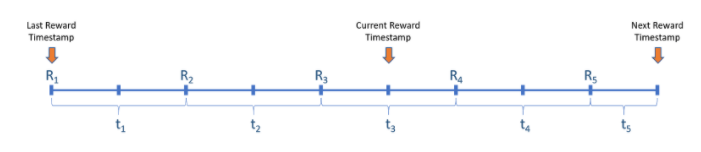# Moving Reward Rate¶

pSTAKE smart contracts have the ability to update the reward rate (staking rewards paid out to stakers) to match the reward rate of the native PoS chain. In case of scenarios like native chain (Cosmos) undergoing maintenance or temporarily being suspended, or a change in the protocol leading to sudden fluctuation in the PoS rewards, there could be discrepancies if the users have not redeemed the reward using the earlier reward rate. Hence, in order to address this inconsistency, pSTAKE adopts a moving reward rate logic, which ensures that the reward rate is updated to match the native chain protocol’s reward rate, the non-redeemed rewards of users are calculated using these multiple reward rates, as per their weighted time interval.

## Arithmetic Calculations Involved¶

The reward rate is not a single value that is set, but an array of values. Every time a new reward rate needs to be set; it is pushed in the reward rate array. Also, the timestamp of the new reward rate setting is pushed in a separate array. Now, to calculate the pending rewards accrued, the user addresses’ last reward update timestamp (rewardsTillTimestamp) is compared with the reward rate timestamp array, then for each interval of reward rate timestamps, the simple interest is calculated. The summation of these simple interests provides the value of rewards accrued.

As an example, consider the following as the multiple rate of rewards (R1, R2, R3, R4, R5 ) set over a period of time. The time blocks in between are (t1, t2, t3, t4, t5):If for a particular account, the stkATOM Balance is B1 at ‘Last Reward Timestamp’, and another B1 amount of stkATOM are going to be added at ‘Current Reward Timestamp’, then before adding the stkATOM, the reward recalculation will happen. If the last time the reward was calculated (and credited) when rate was R1 (shown in figure), and if the reward calculation has been triggered again at the marker denoting “Current Reward Timestamp”, then the reward is calculated as:

Reward = B1 x { (R1 x t1) + (R2 x t2) + (R3 x (t3 / 2)) }

The rewardsTillTimestamp value is then updated to the ‘Current Reward Timestamp’ marker. Also, the stkATOM balance is updated with the incoming balance, post crediting of reward, which brings the new stkATOM balance to 2B1. Now again if another reward recalculation is triggered in the ‘Next Reward Timestamp’ marker, then the Rewards are calculated as:

Reward = 2B1 x { ((R3 x (t3 / 2)) + (R4 x t4) + (R5 x t5) }

This ensures that no matter how many times the reward rate is updated, all the values are stored in an array and weighted rewards for each time-block are calculated and credited, thereby ensuring no losses in rewards.